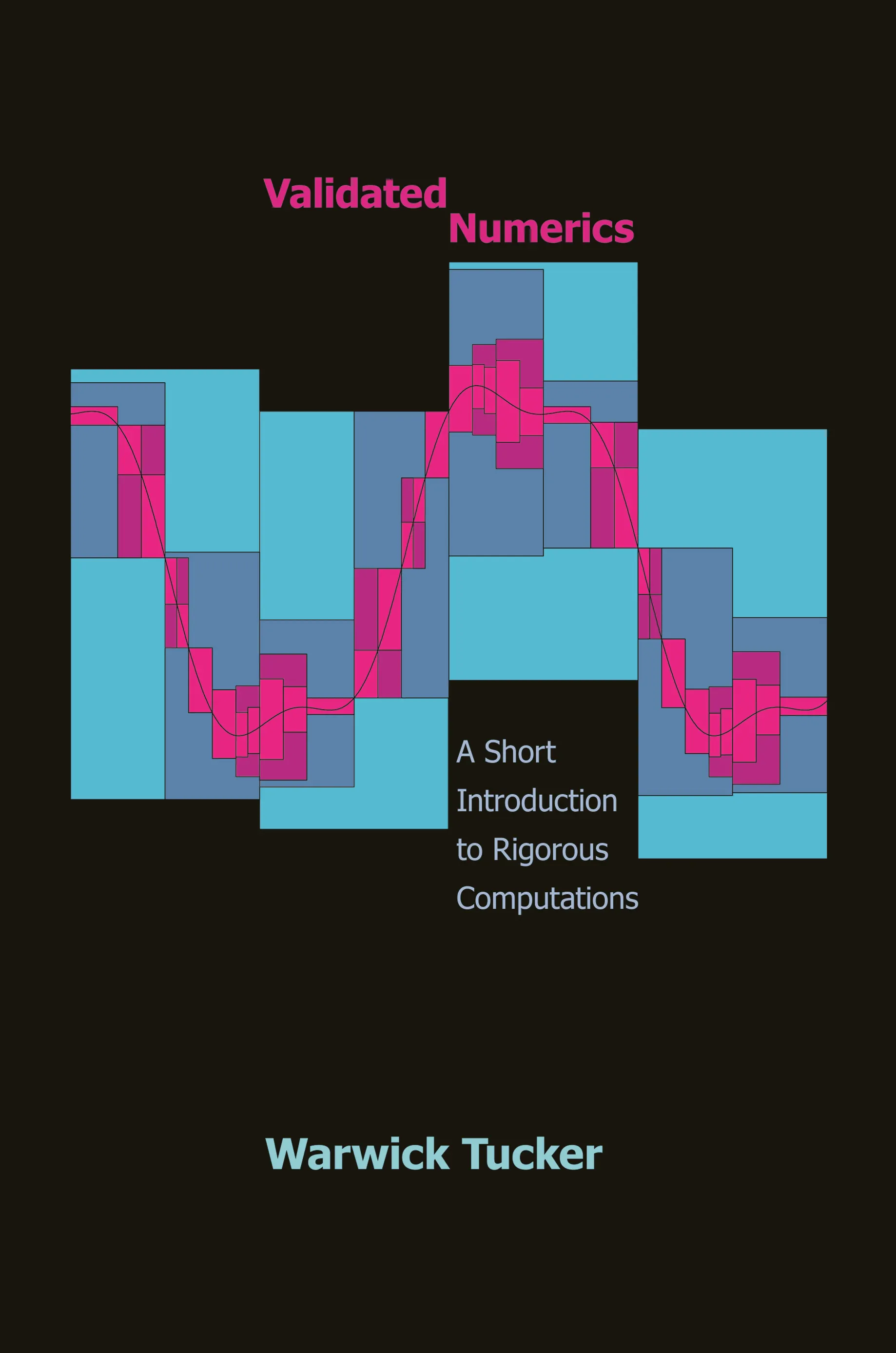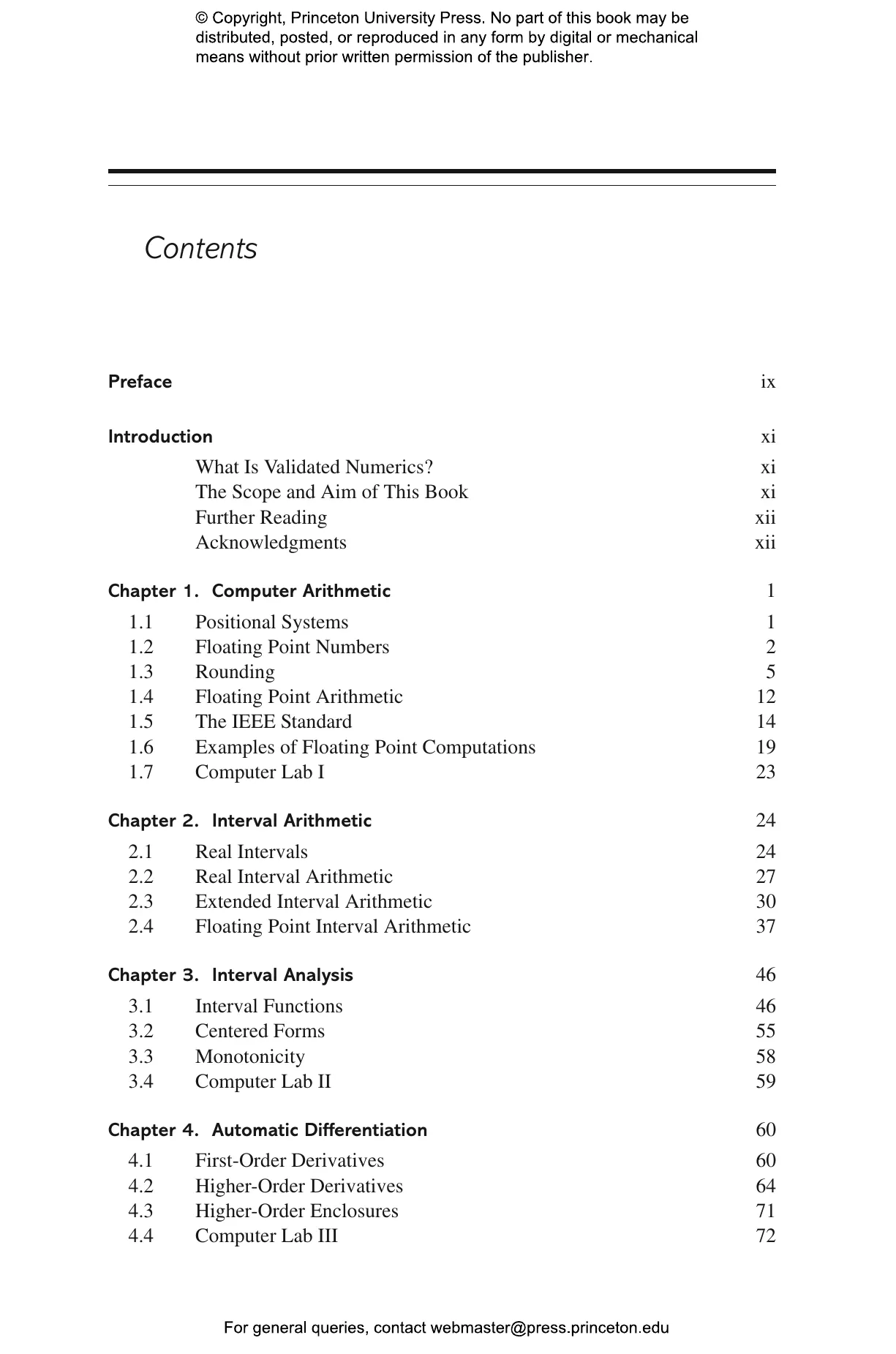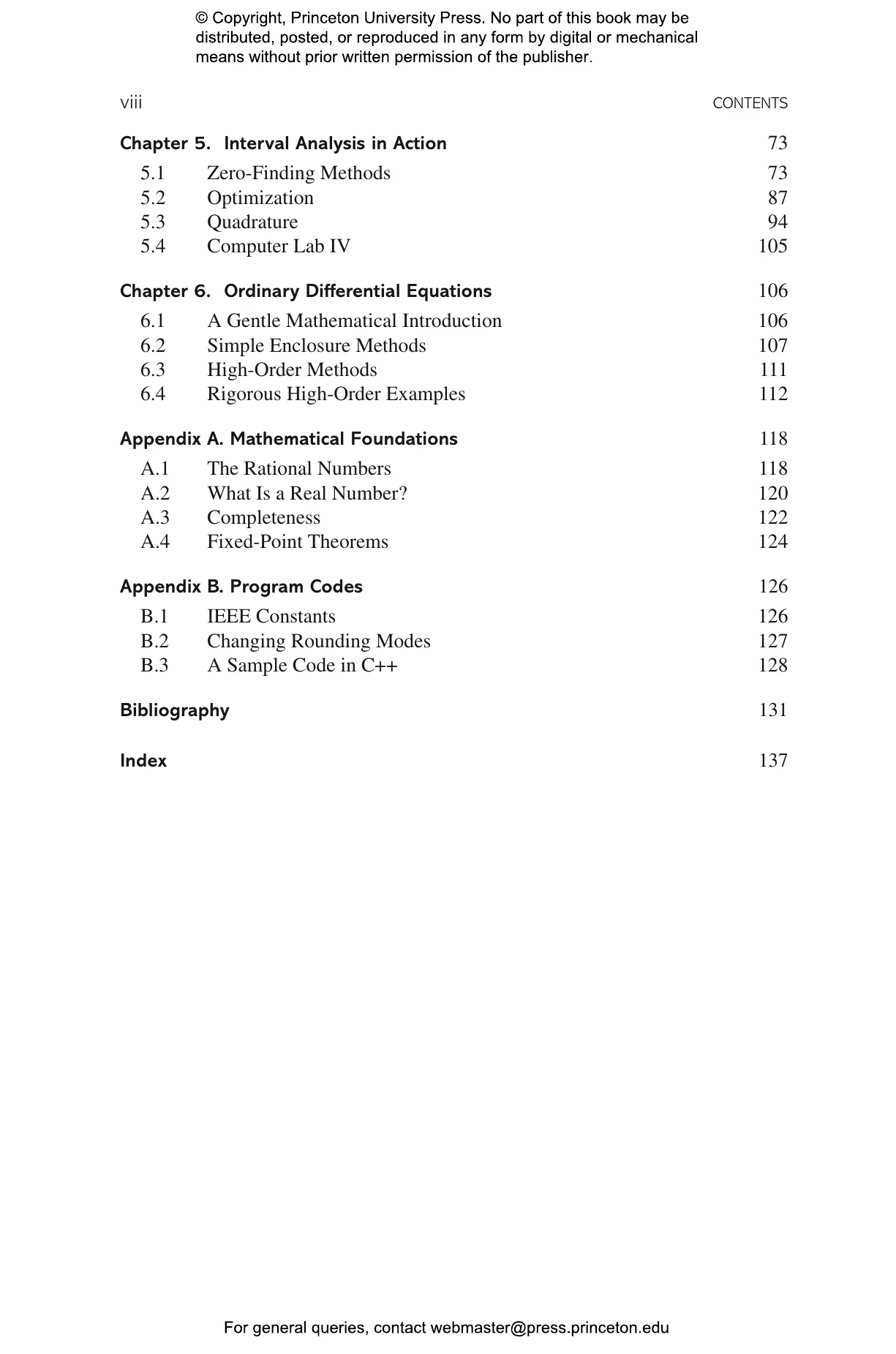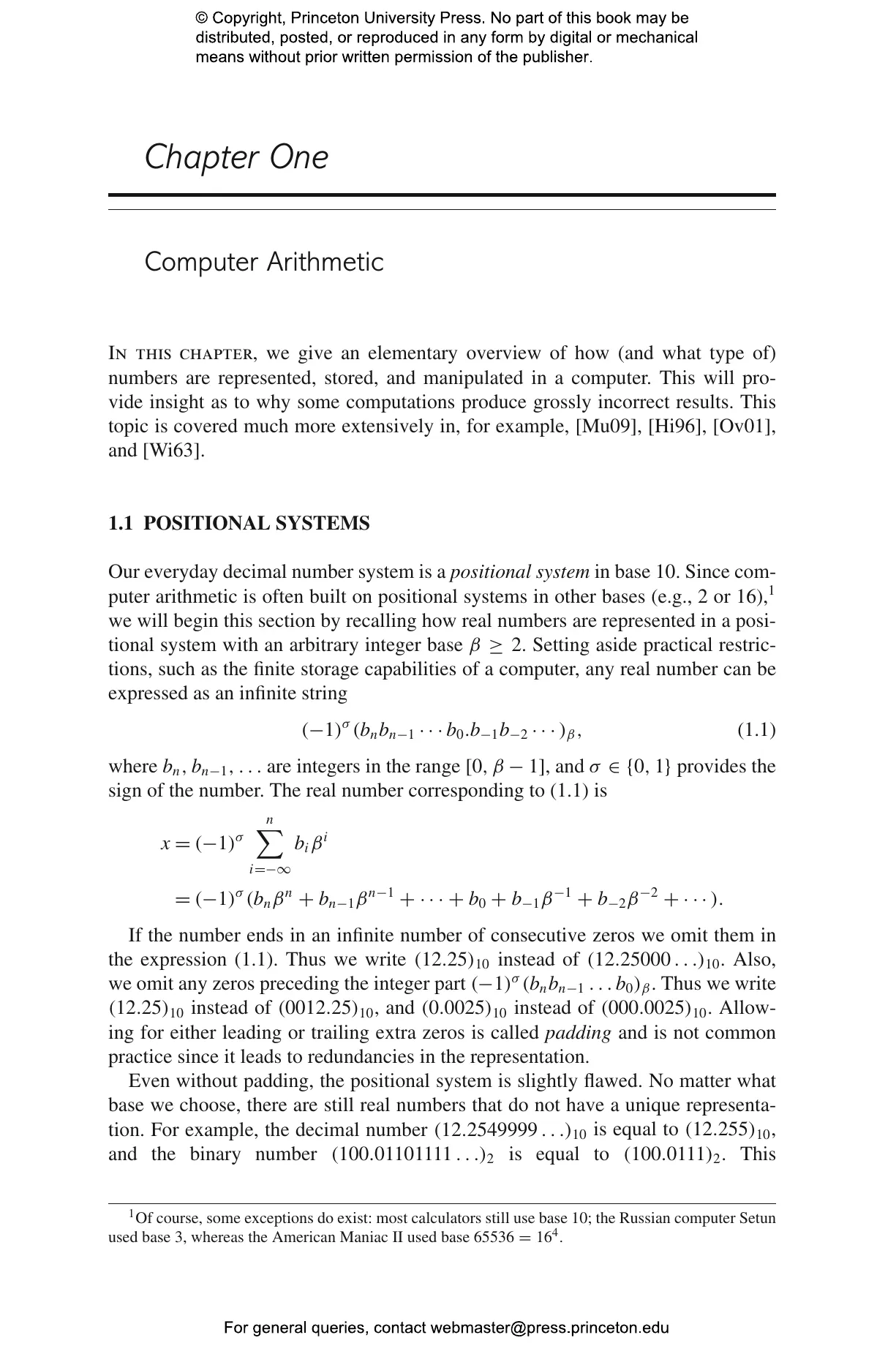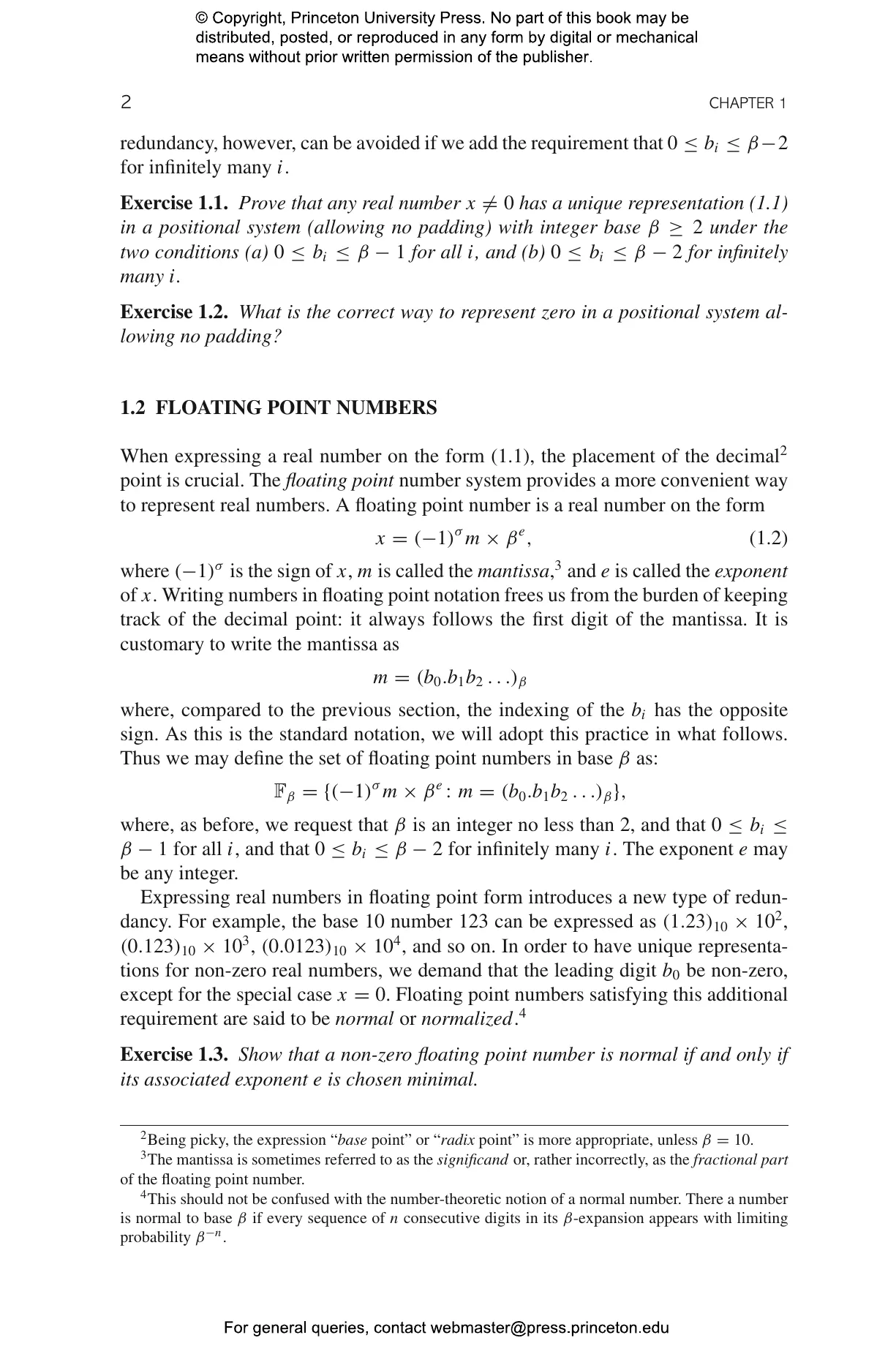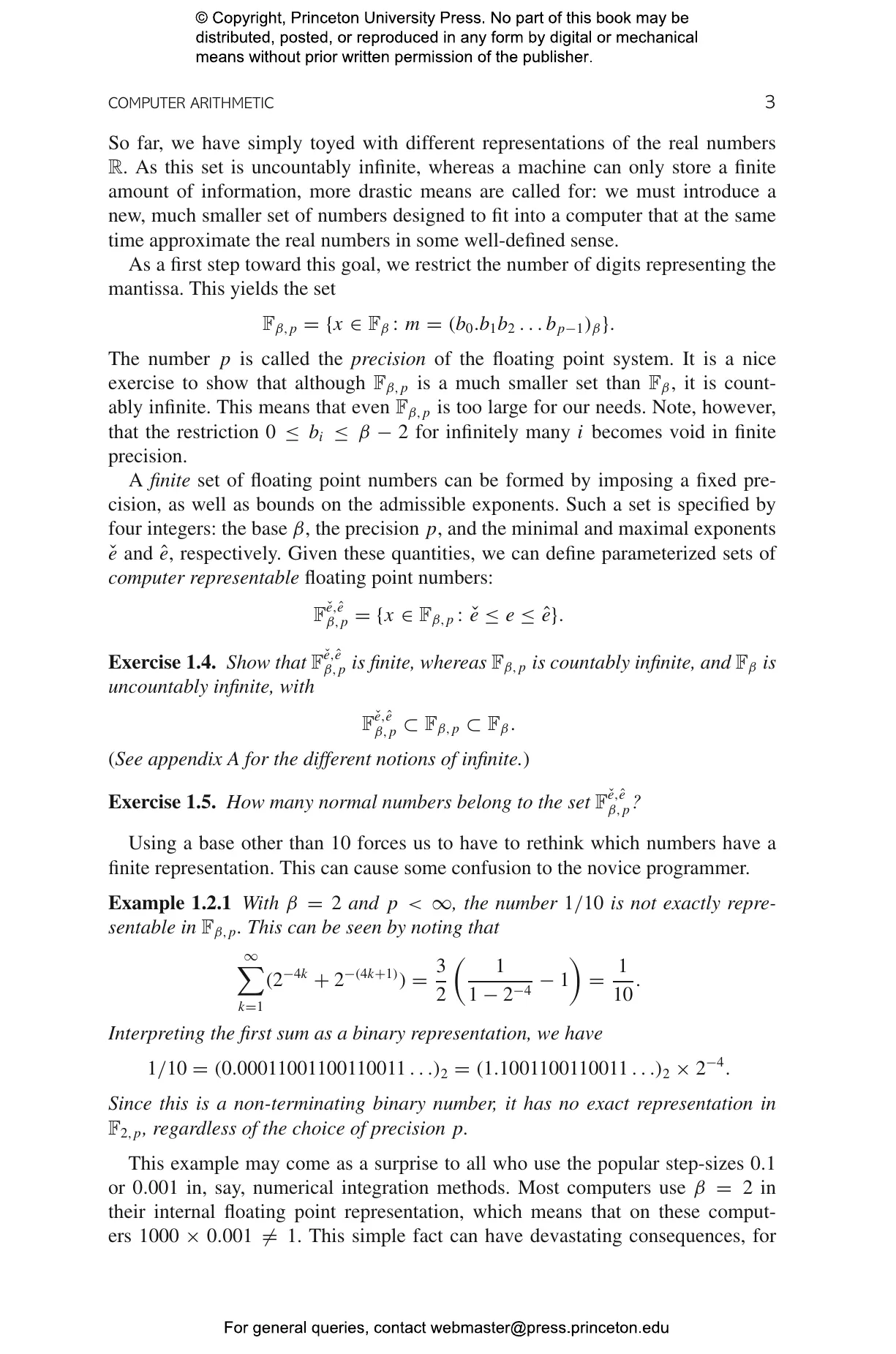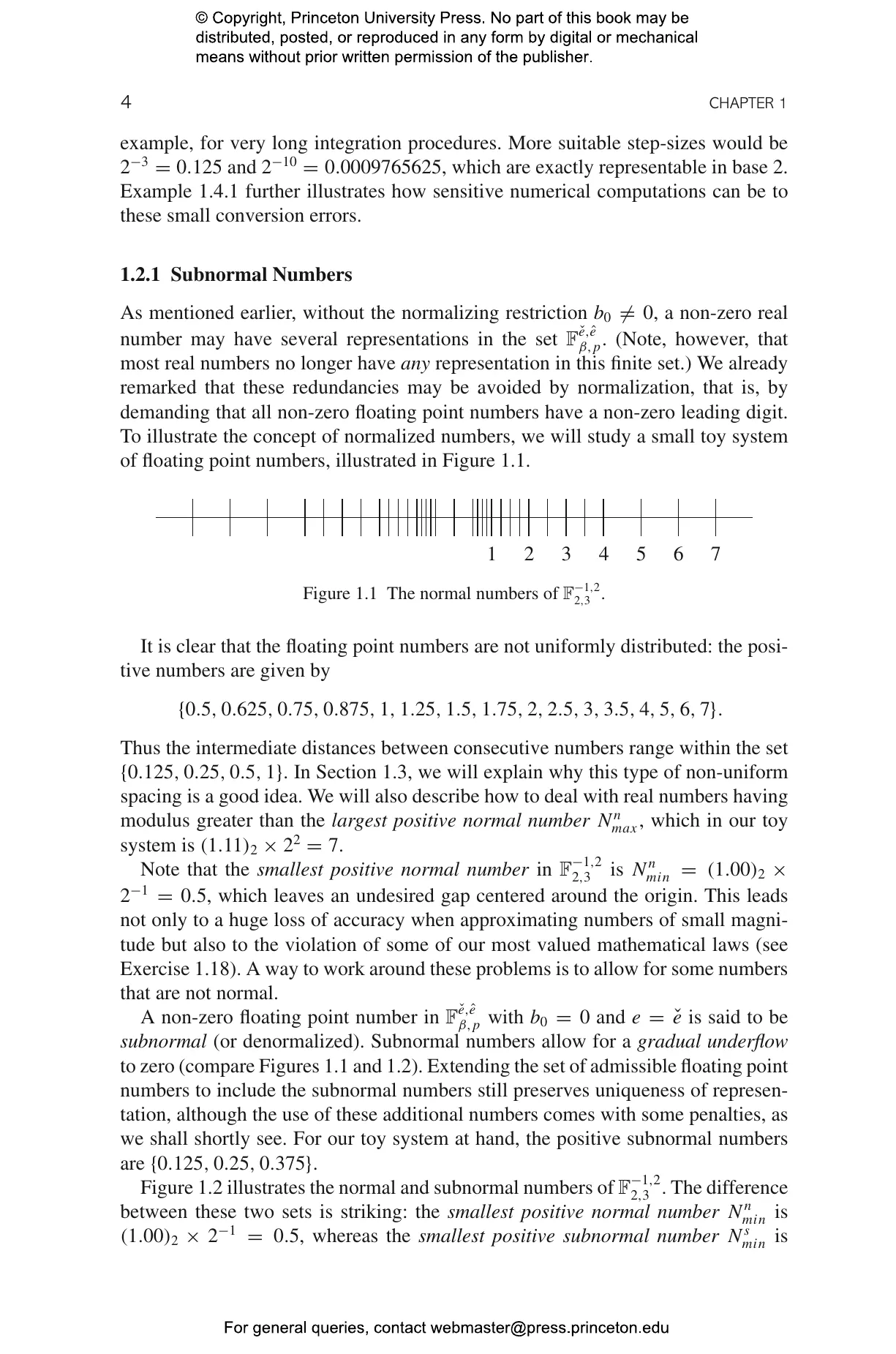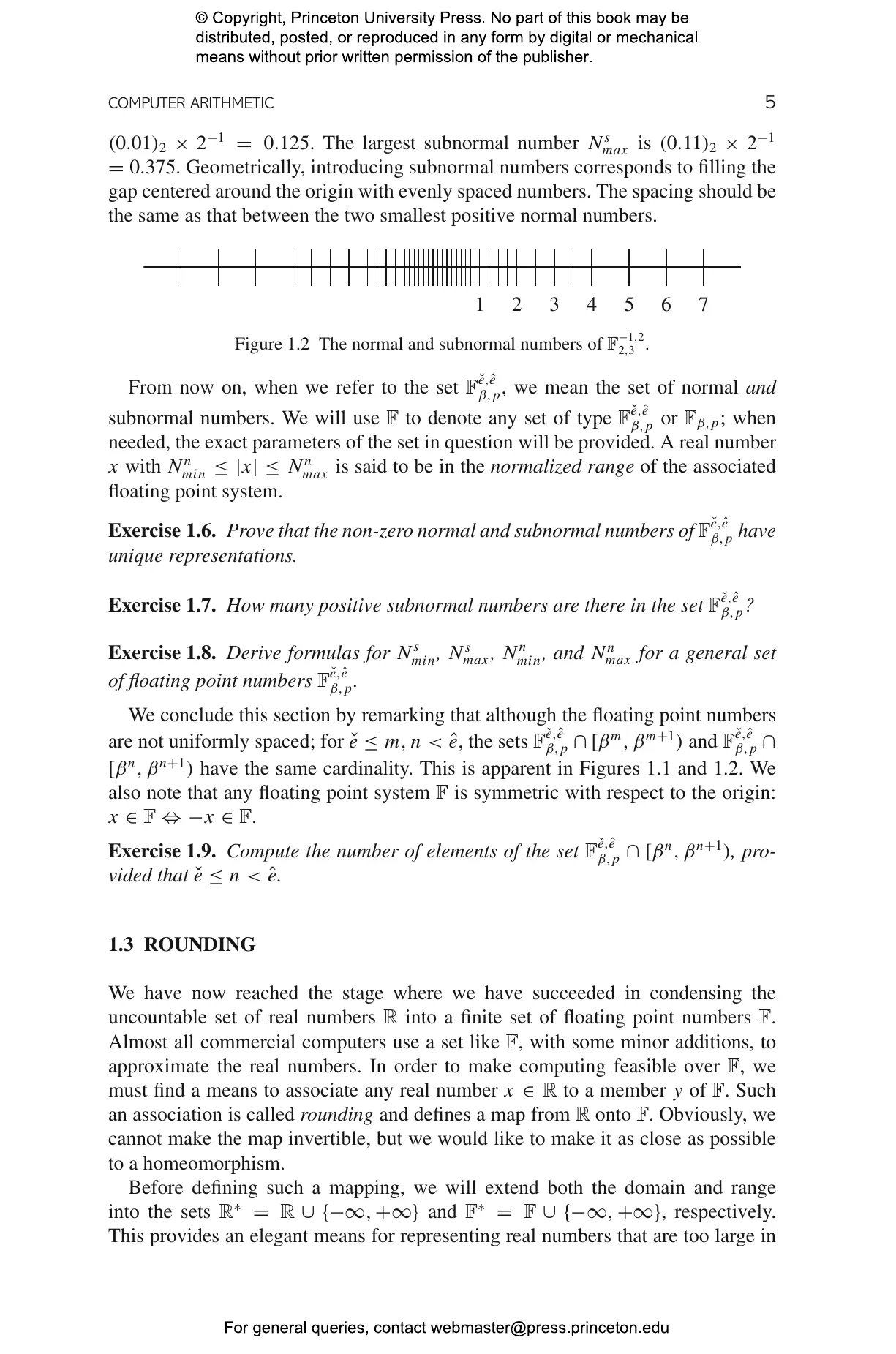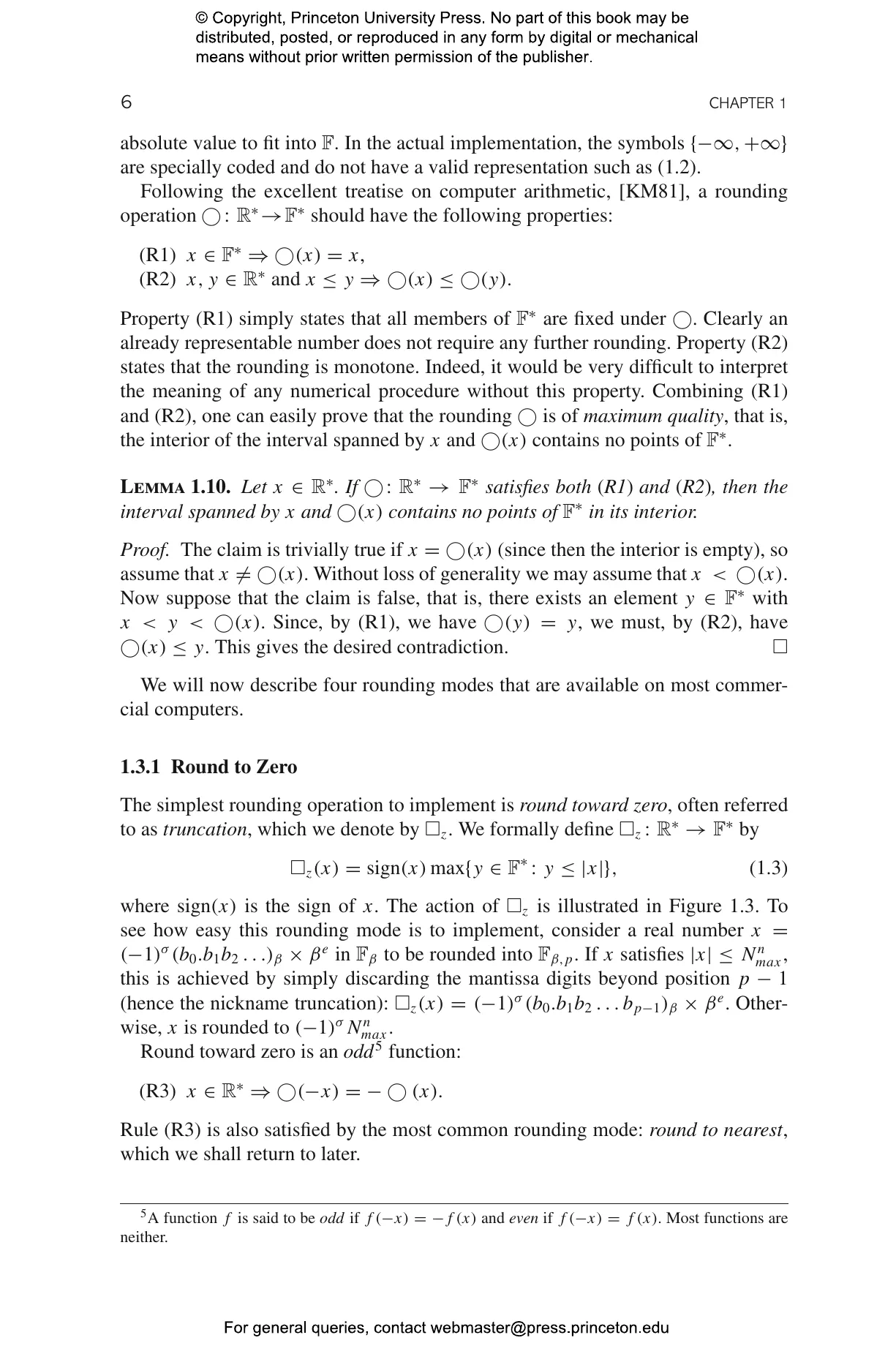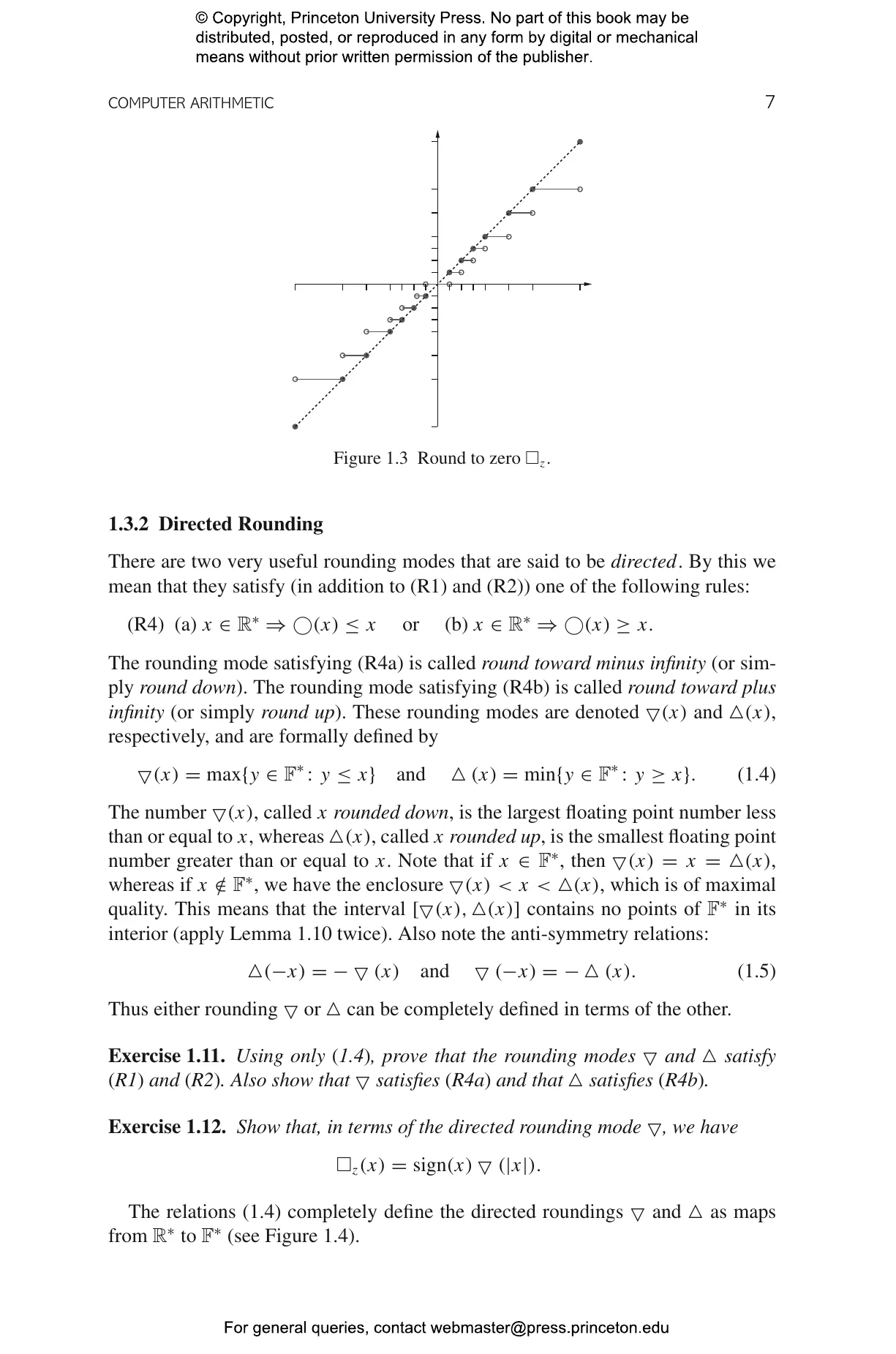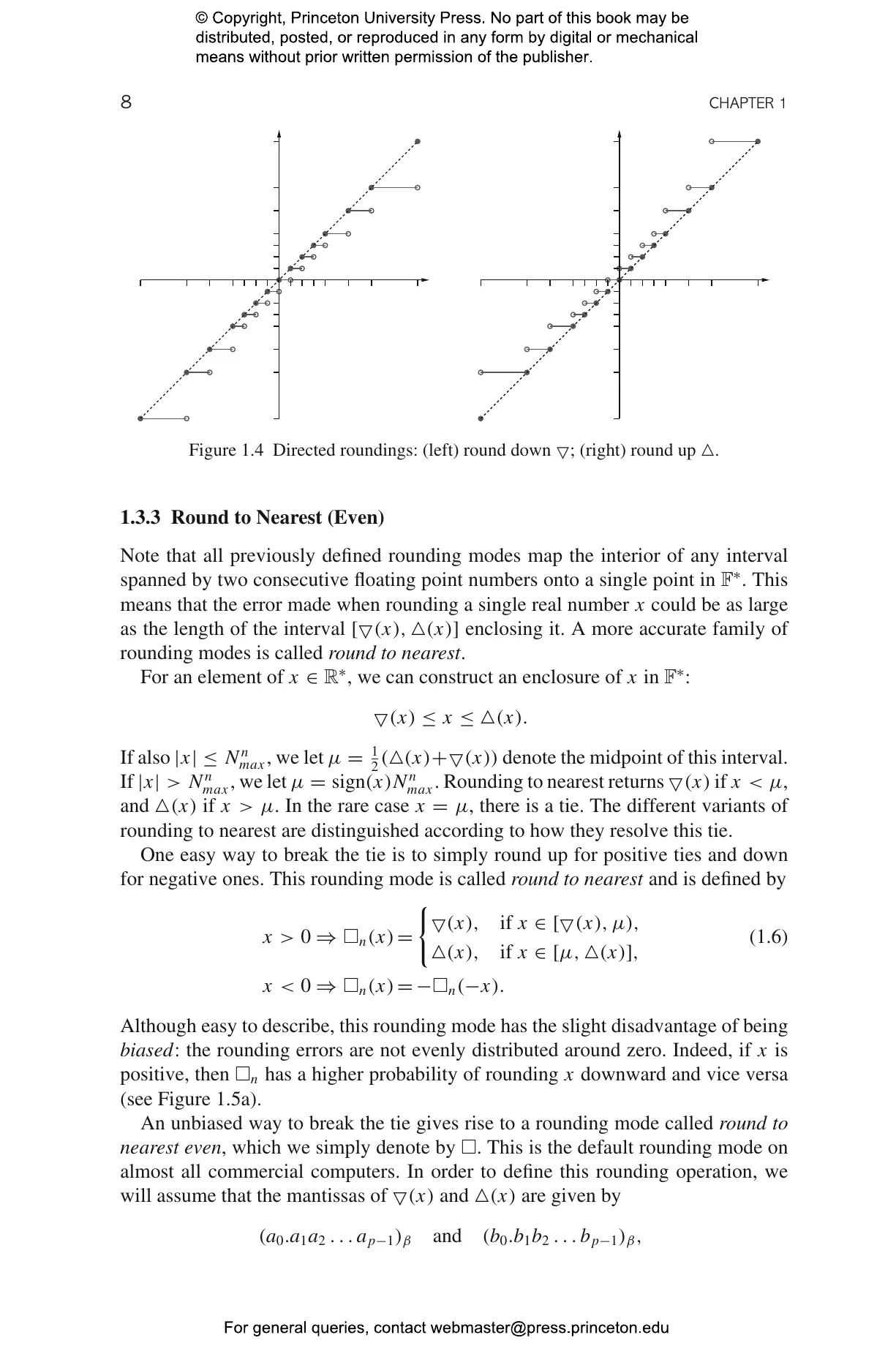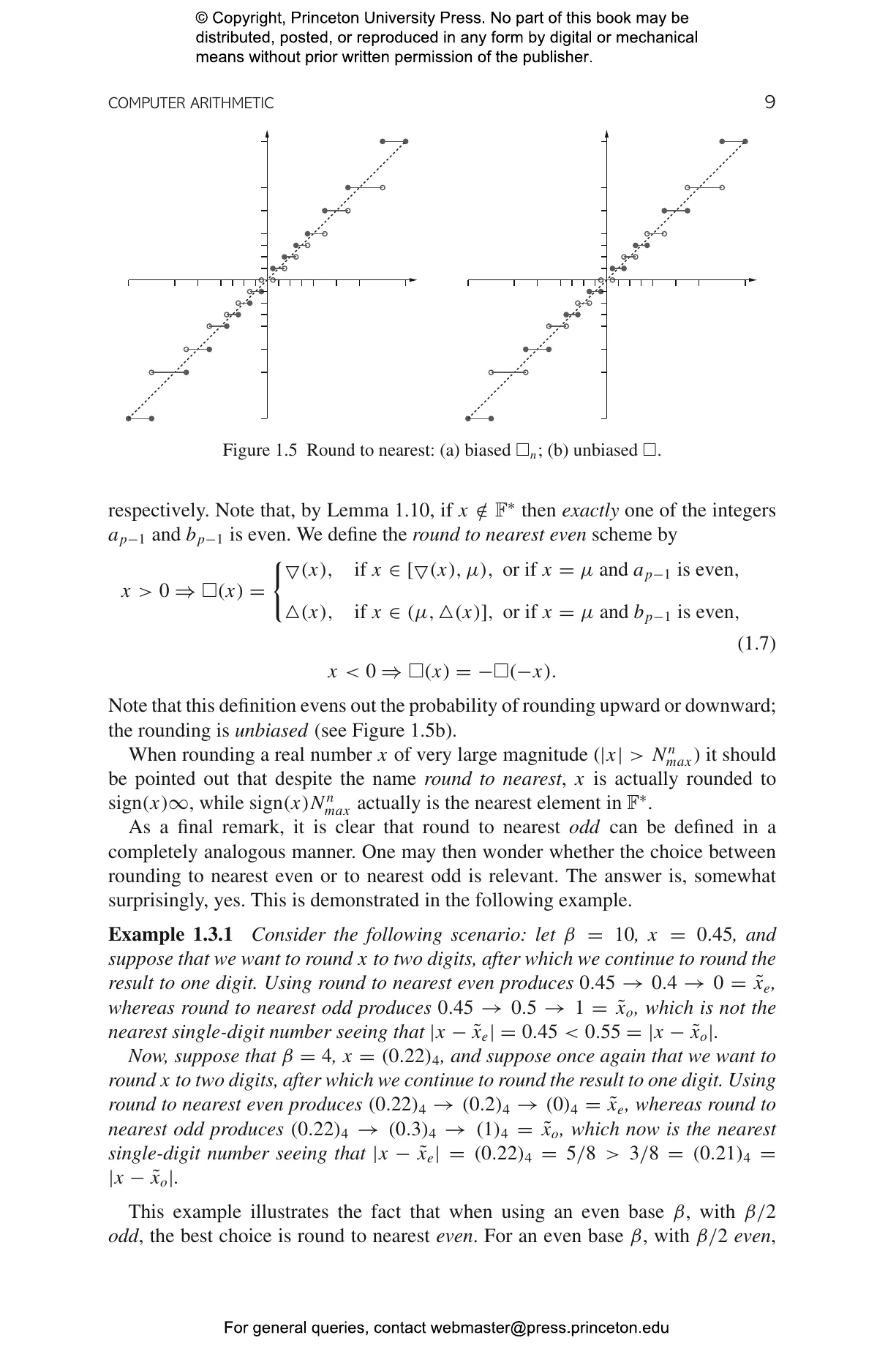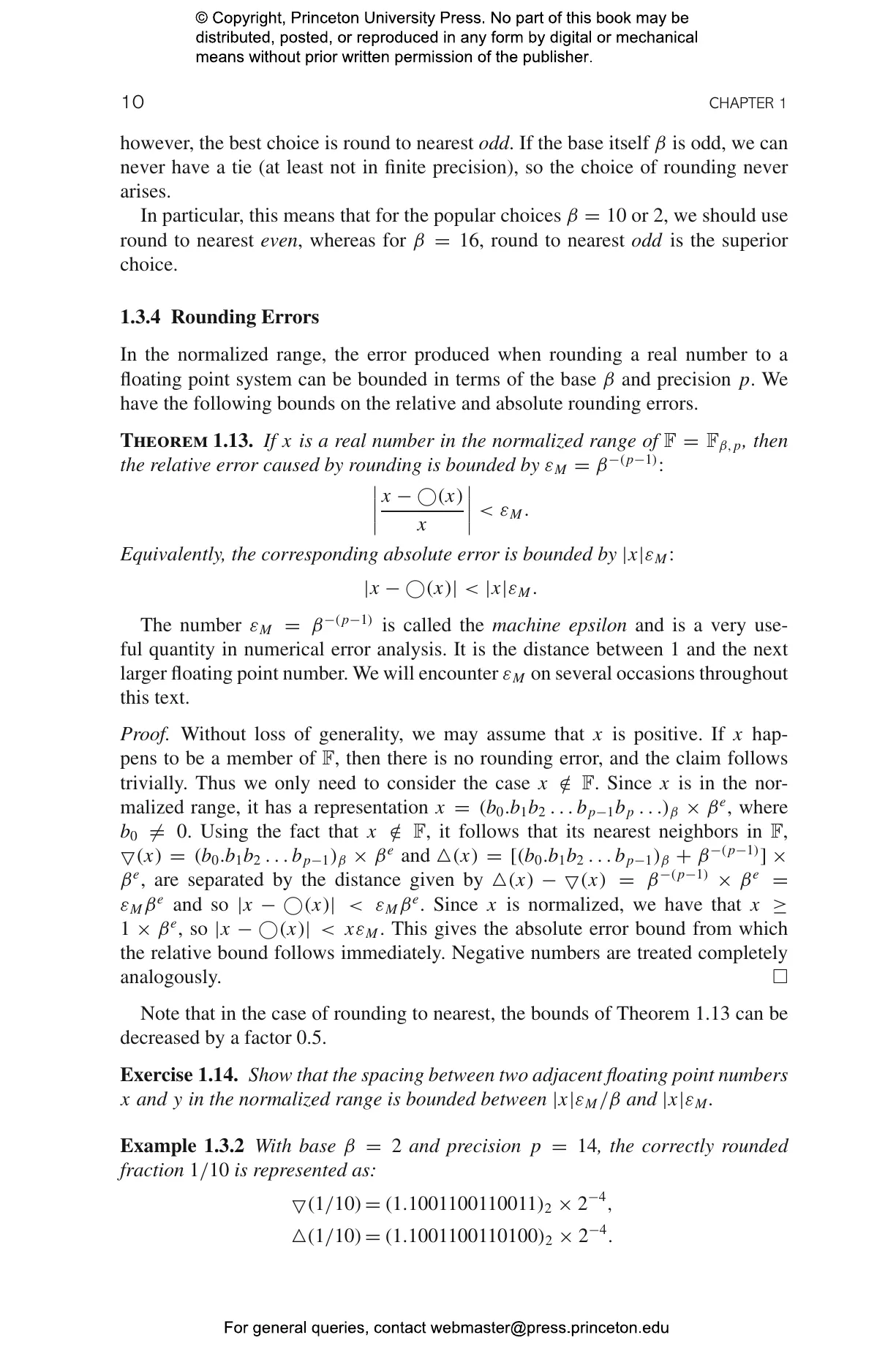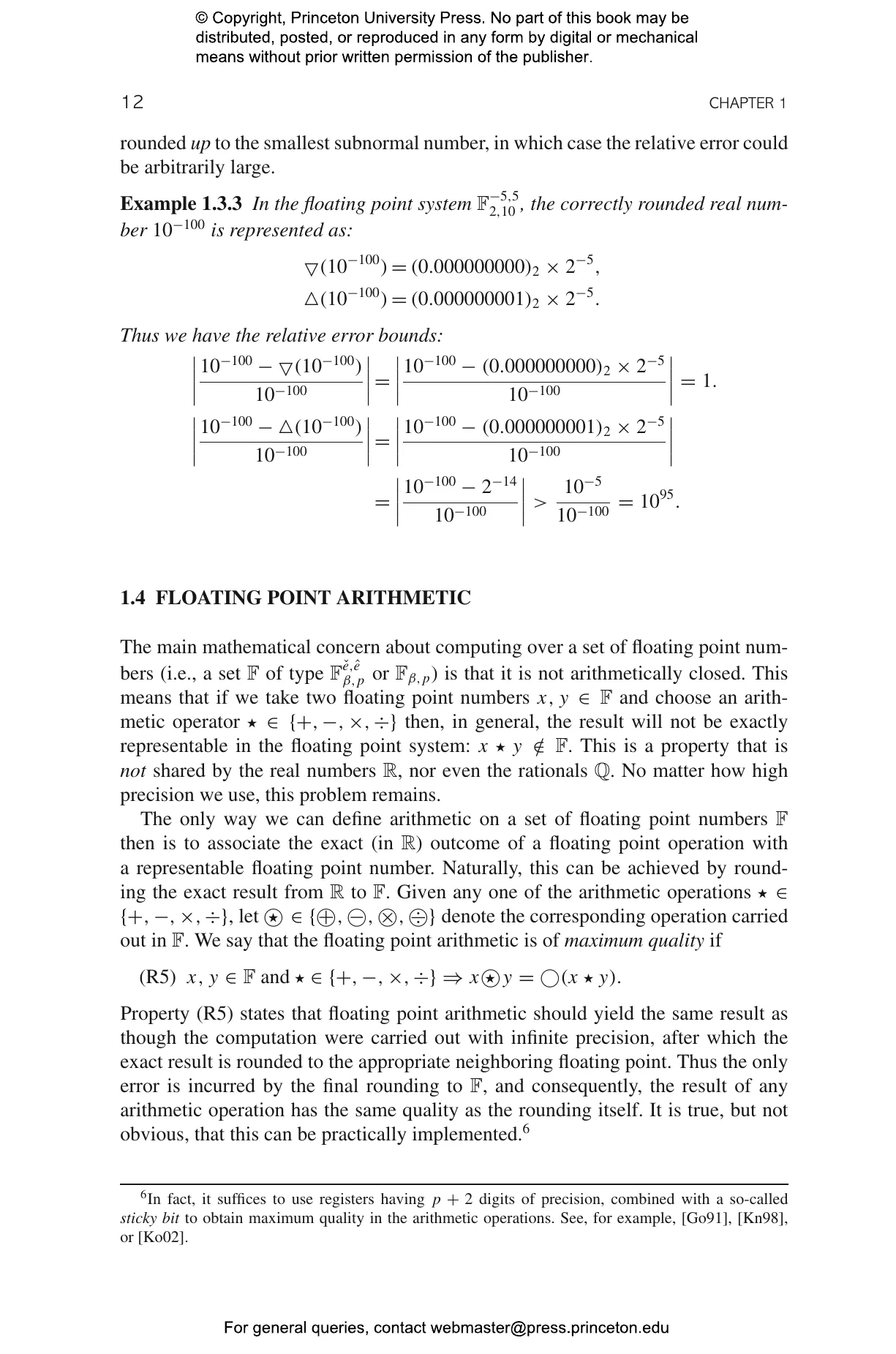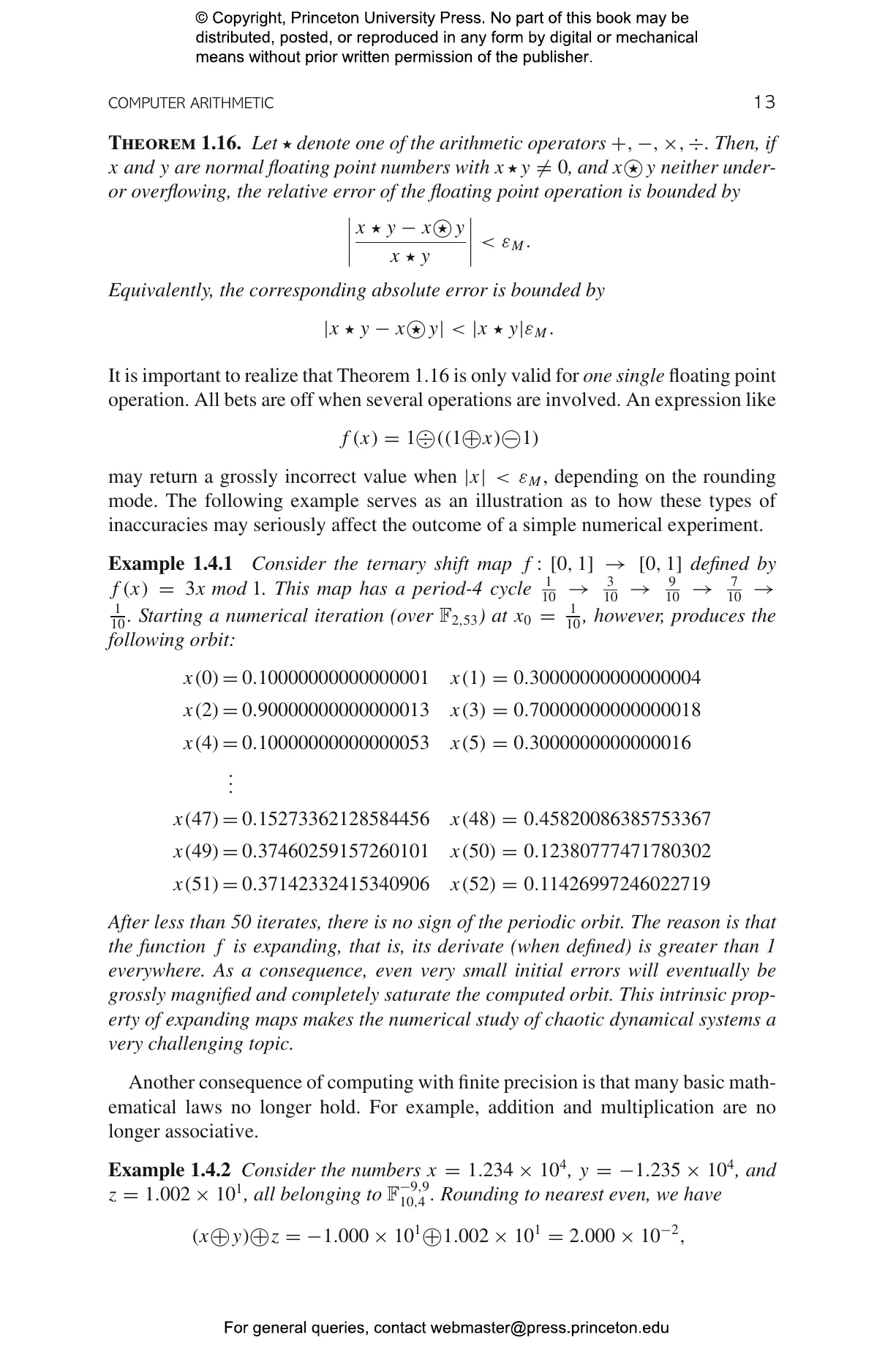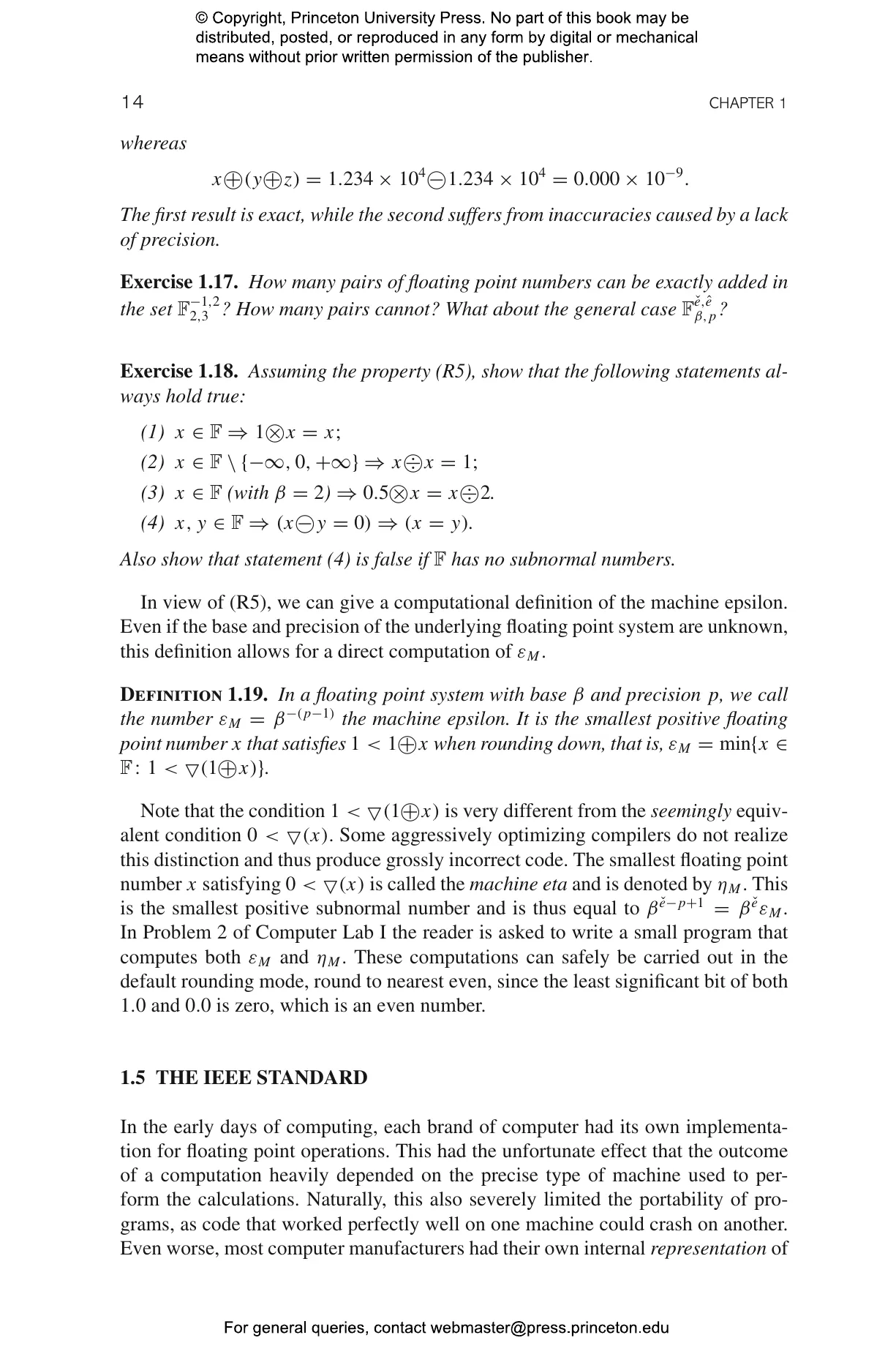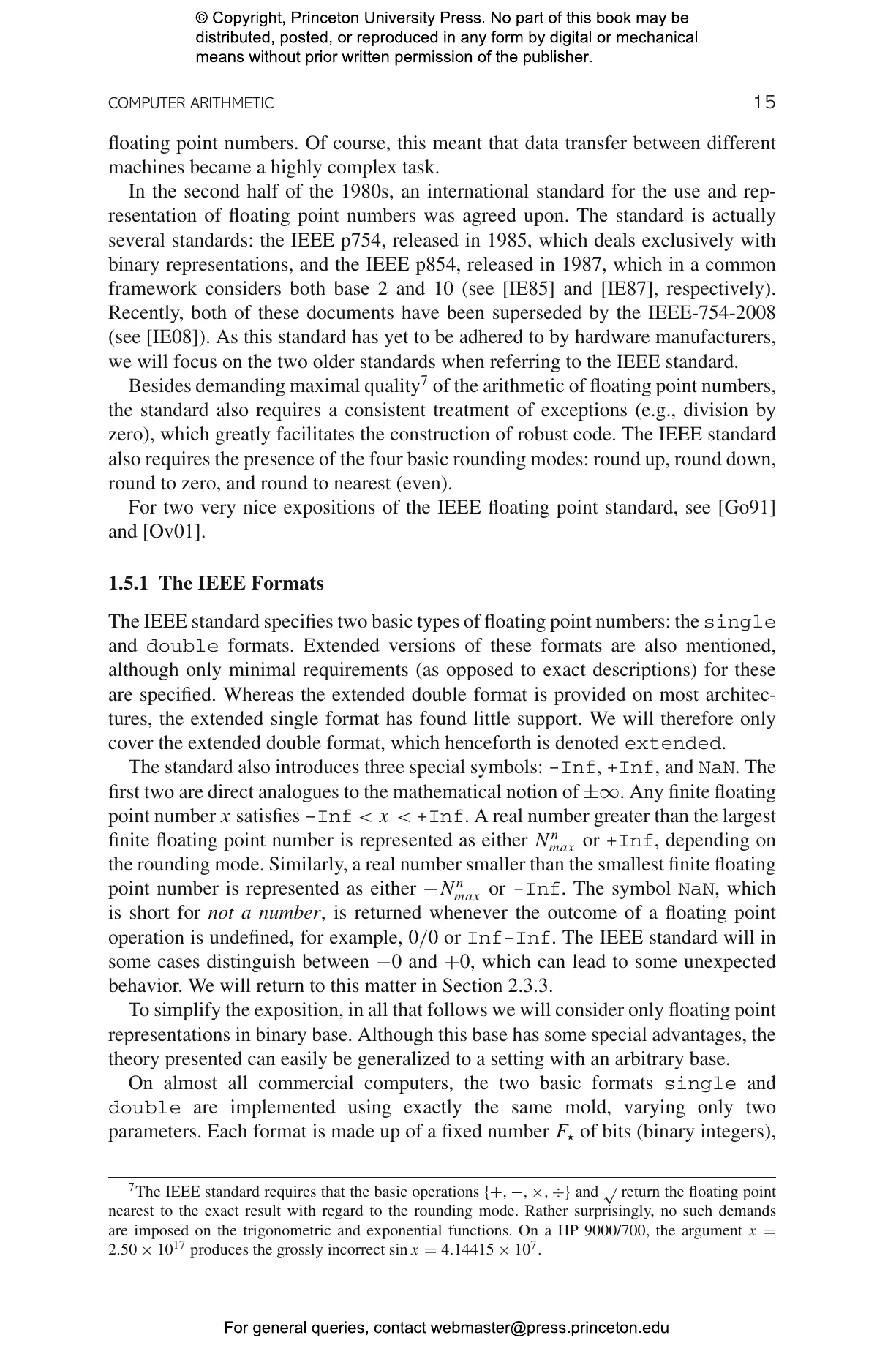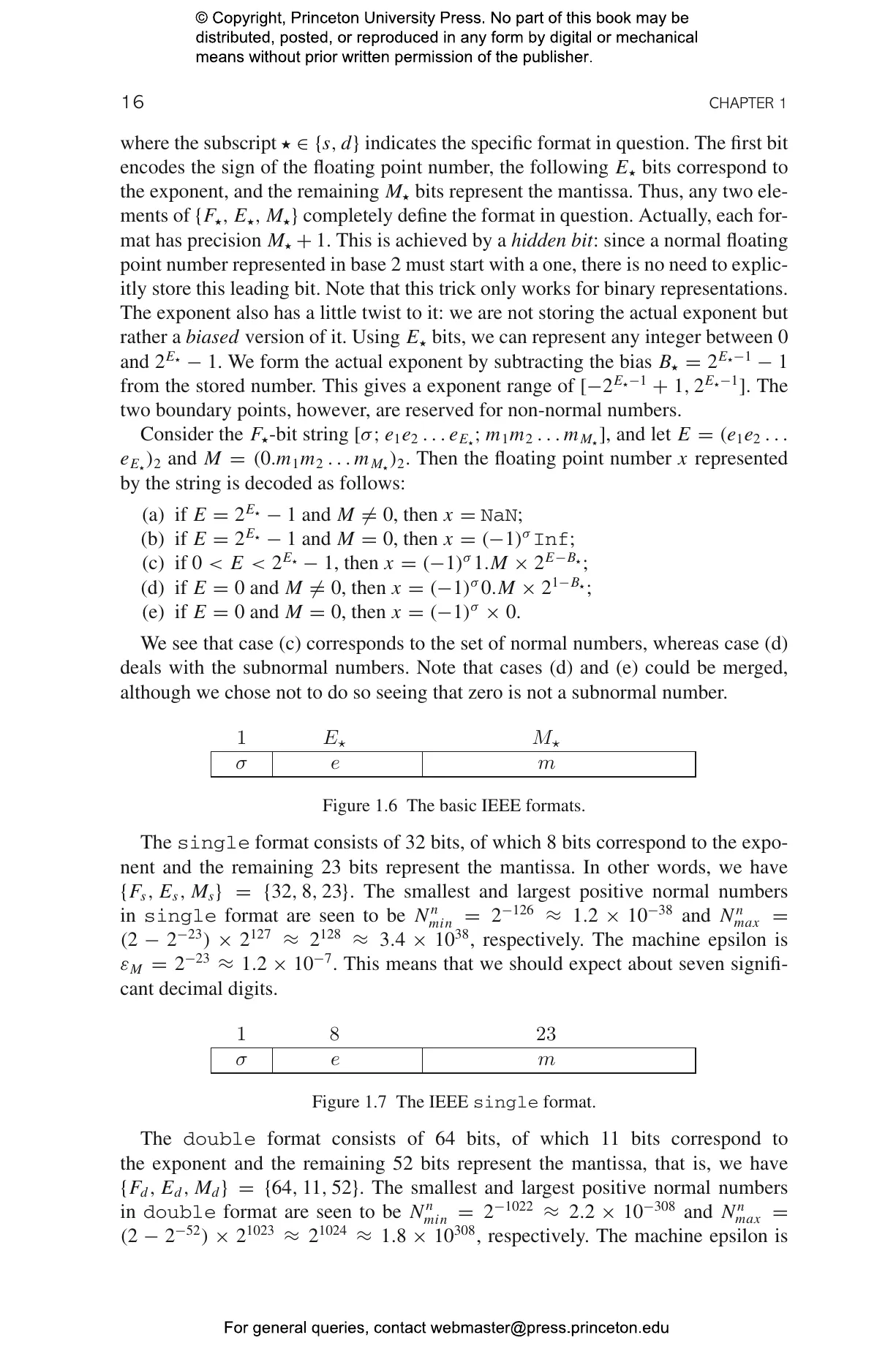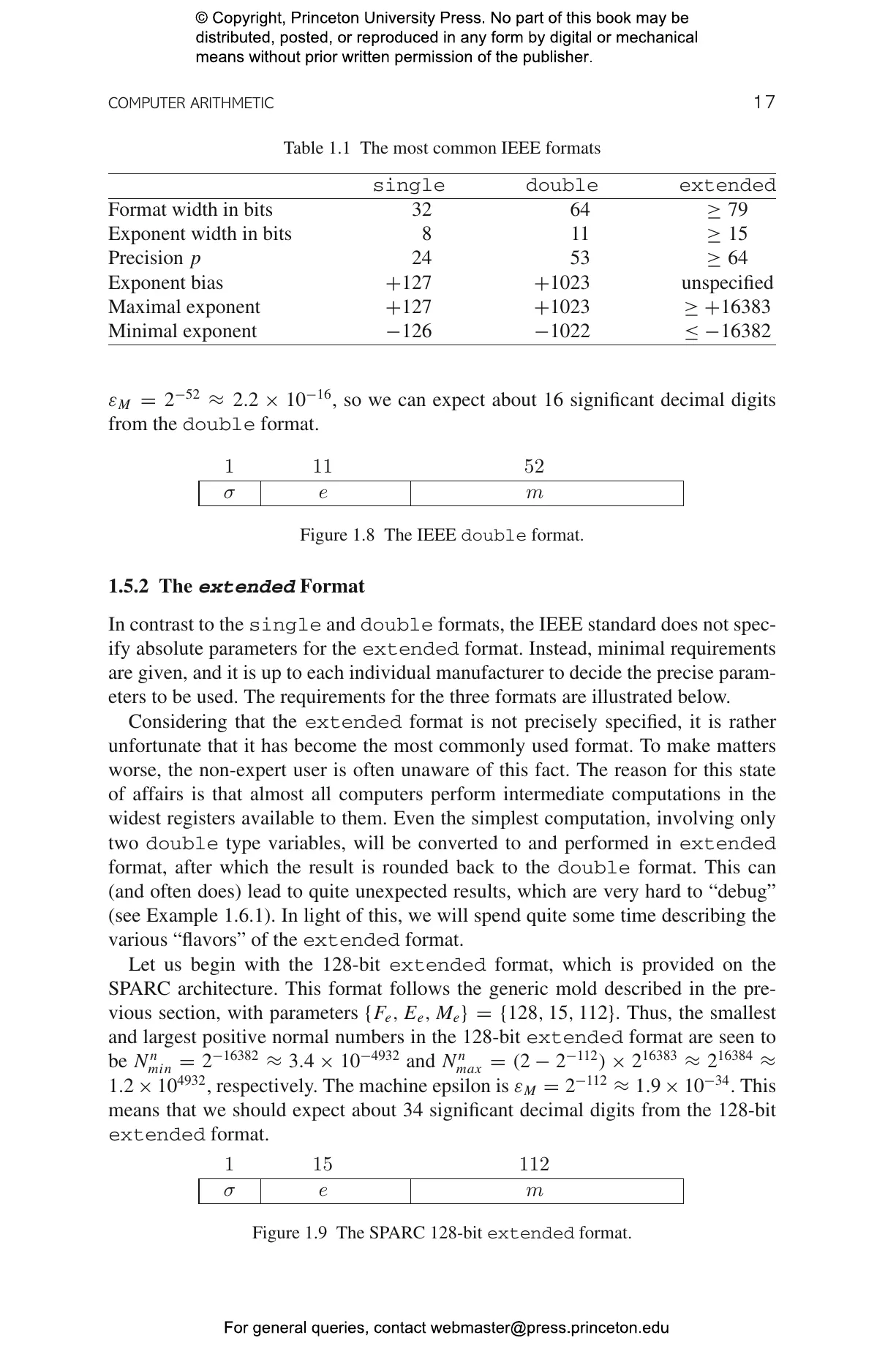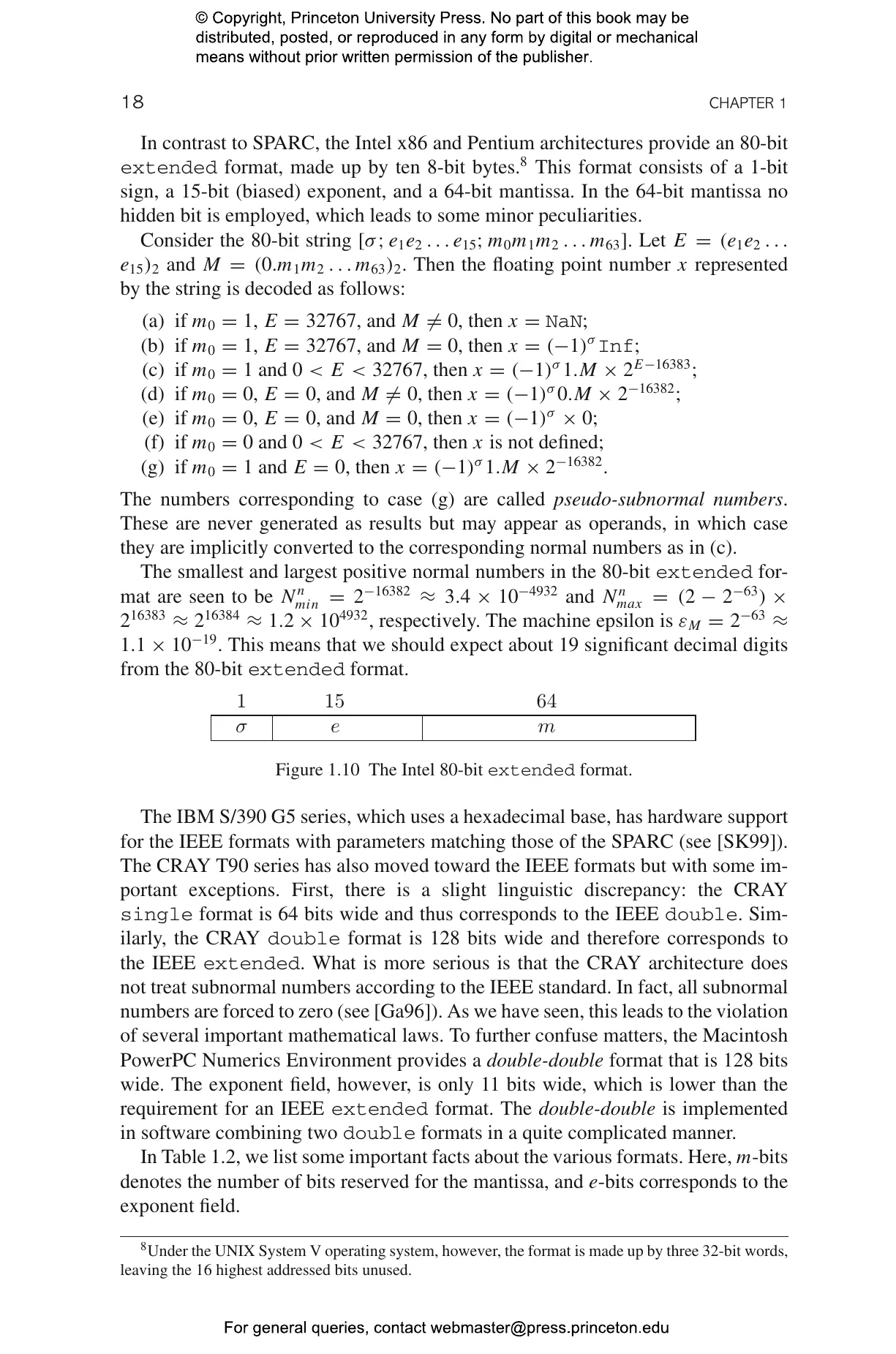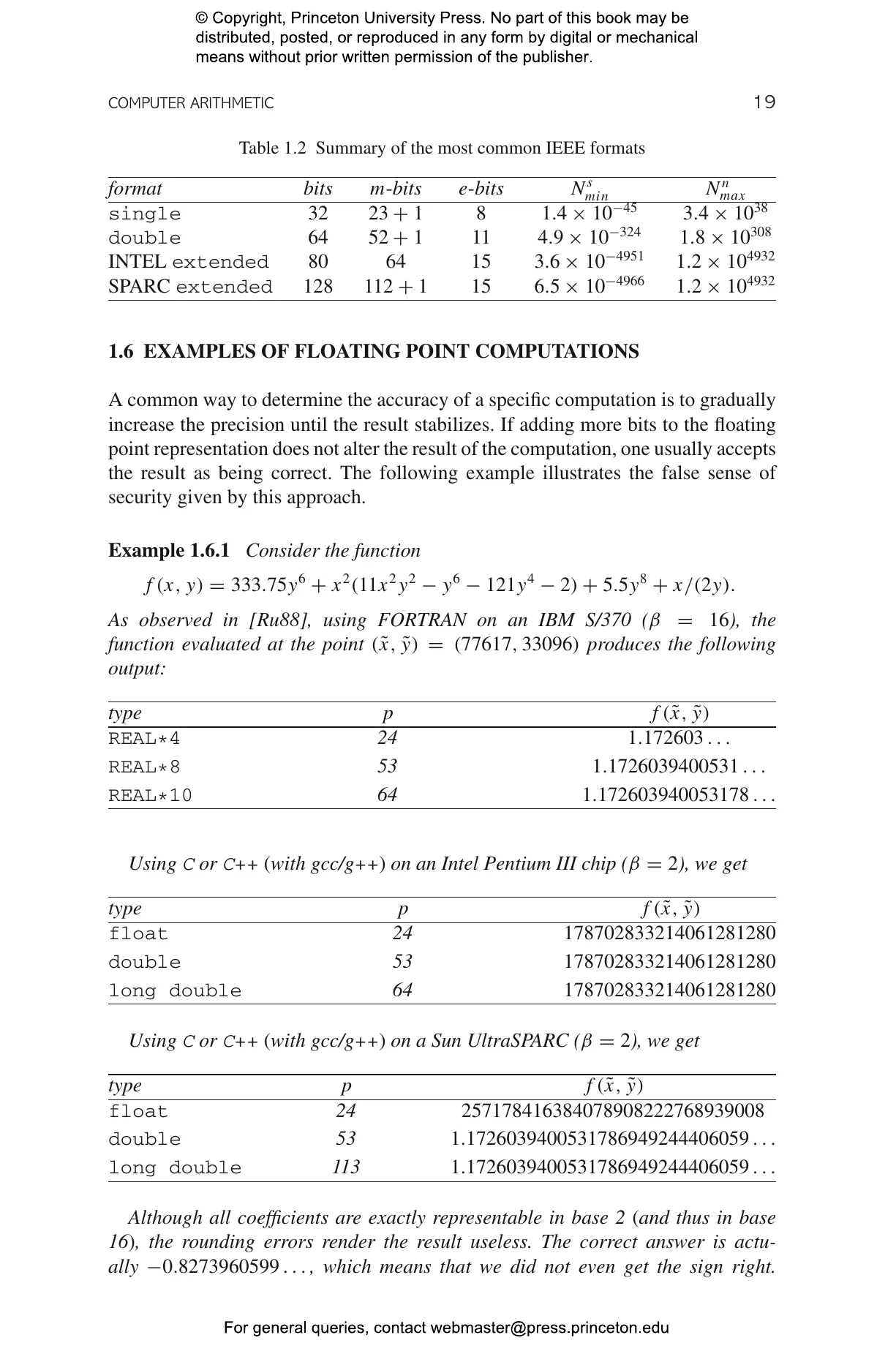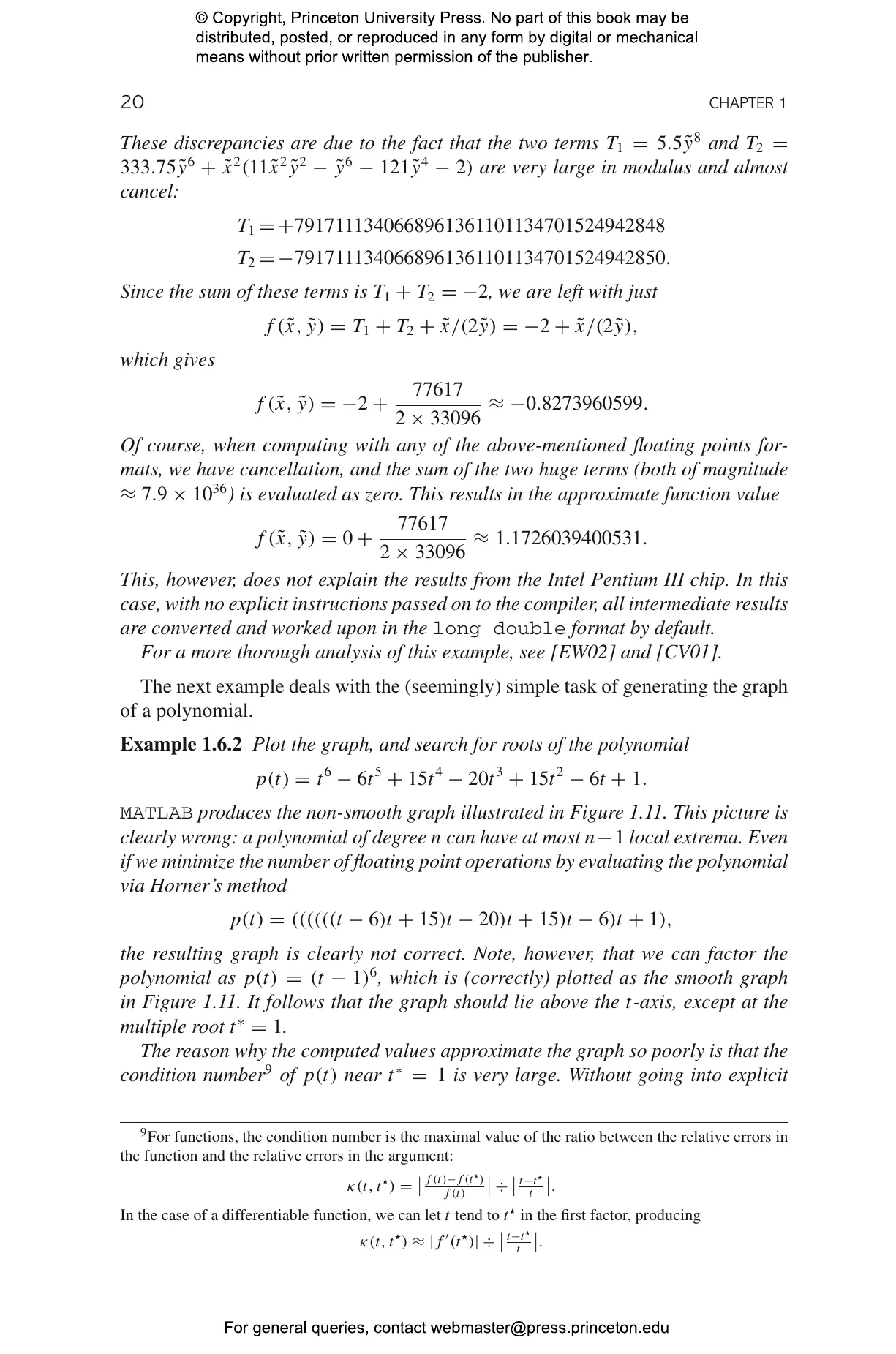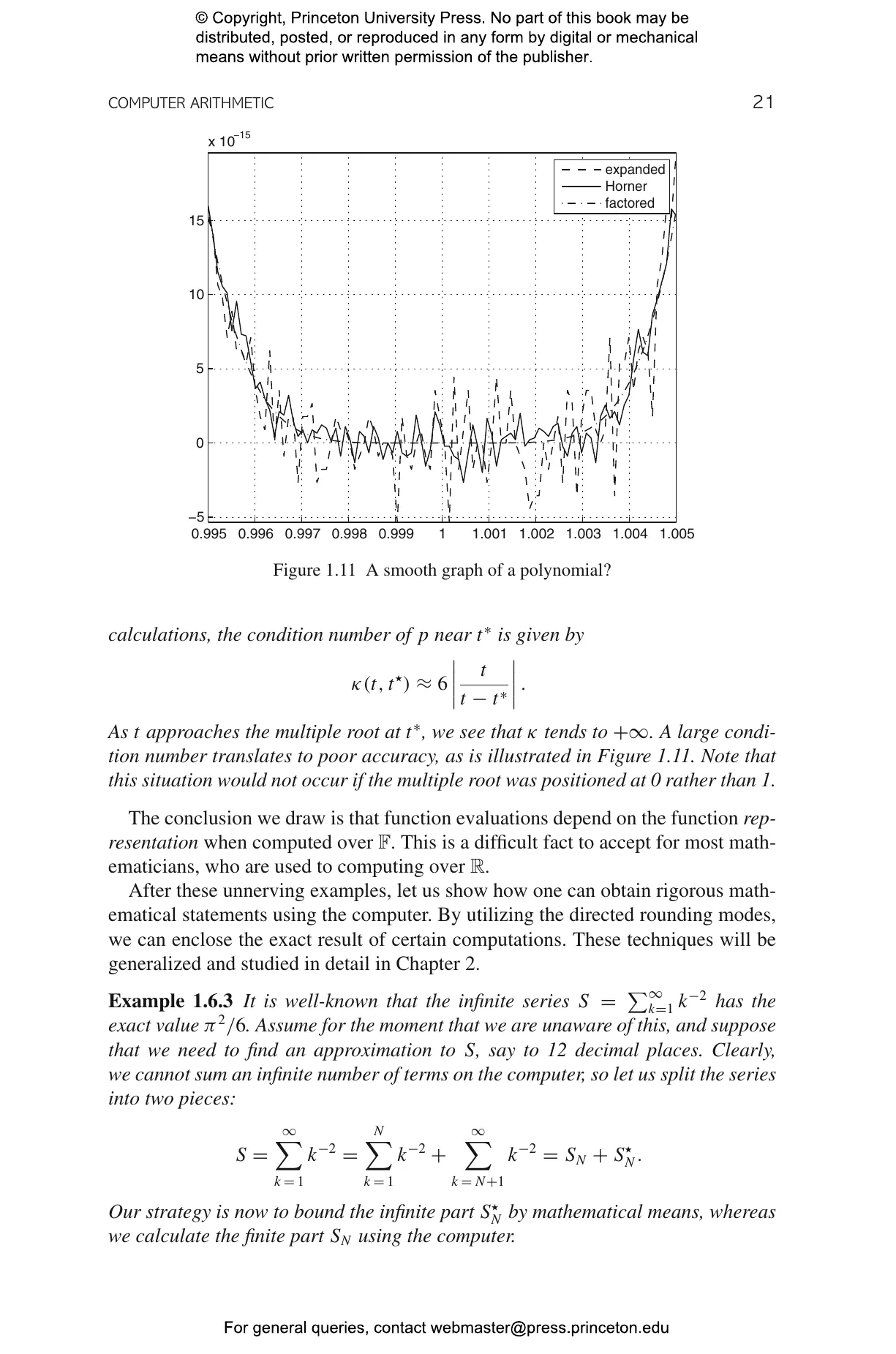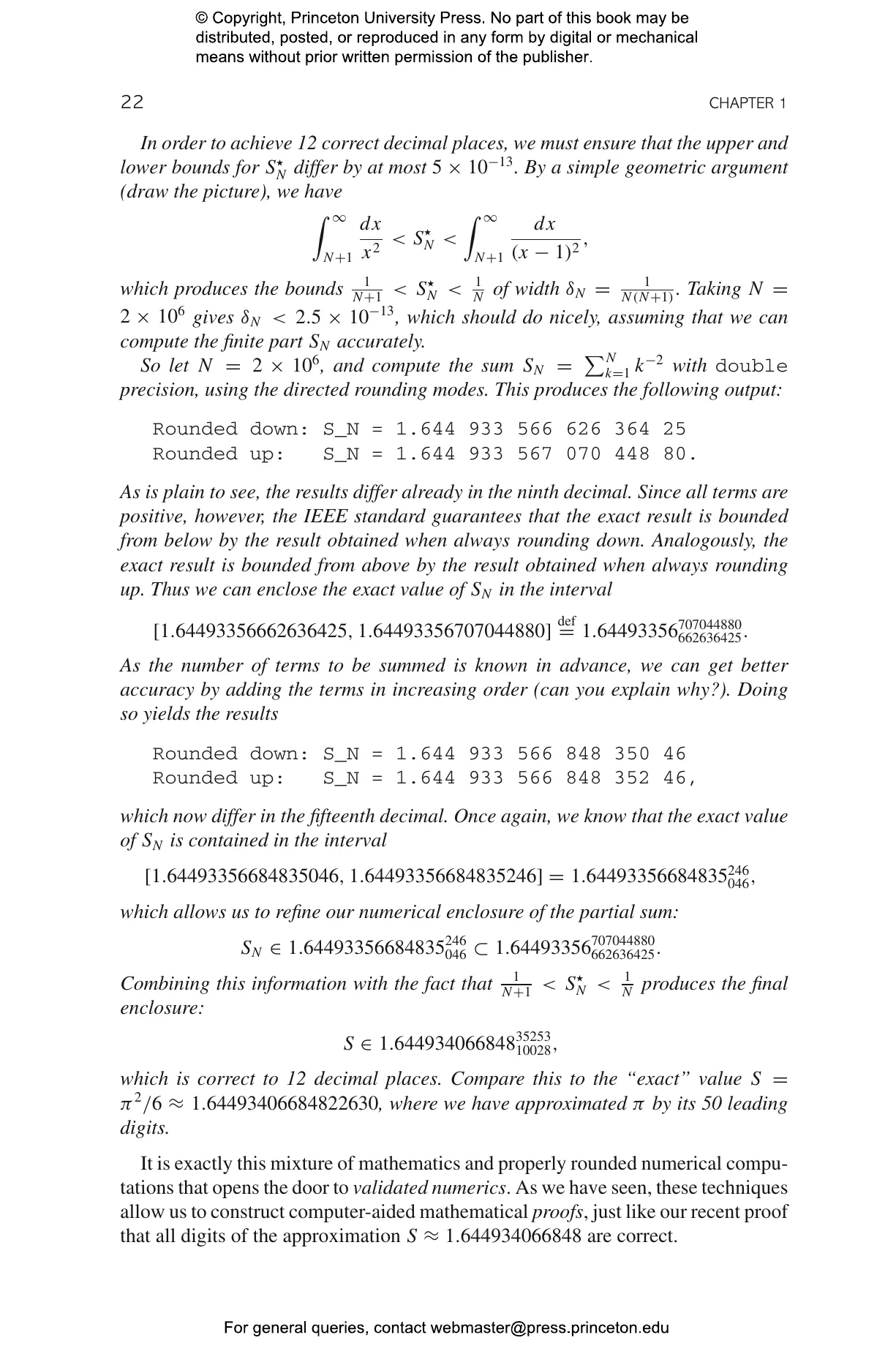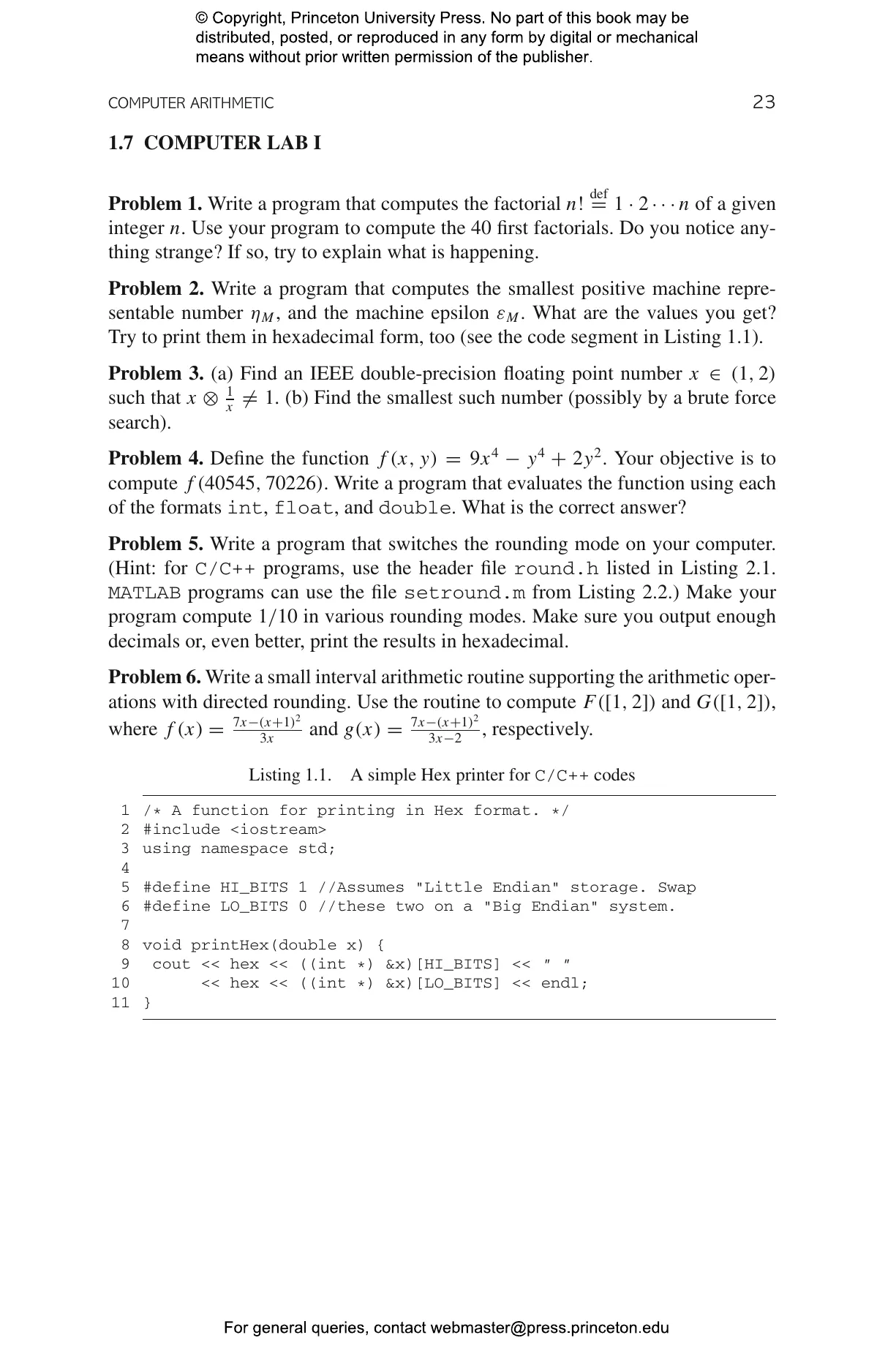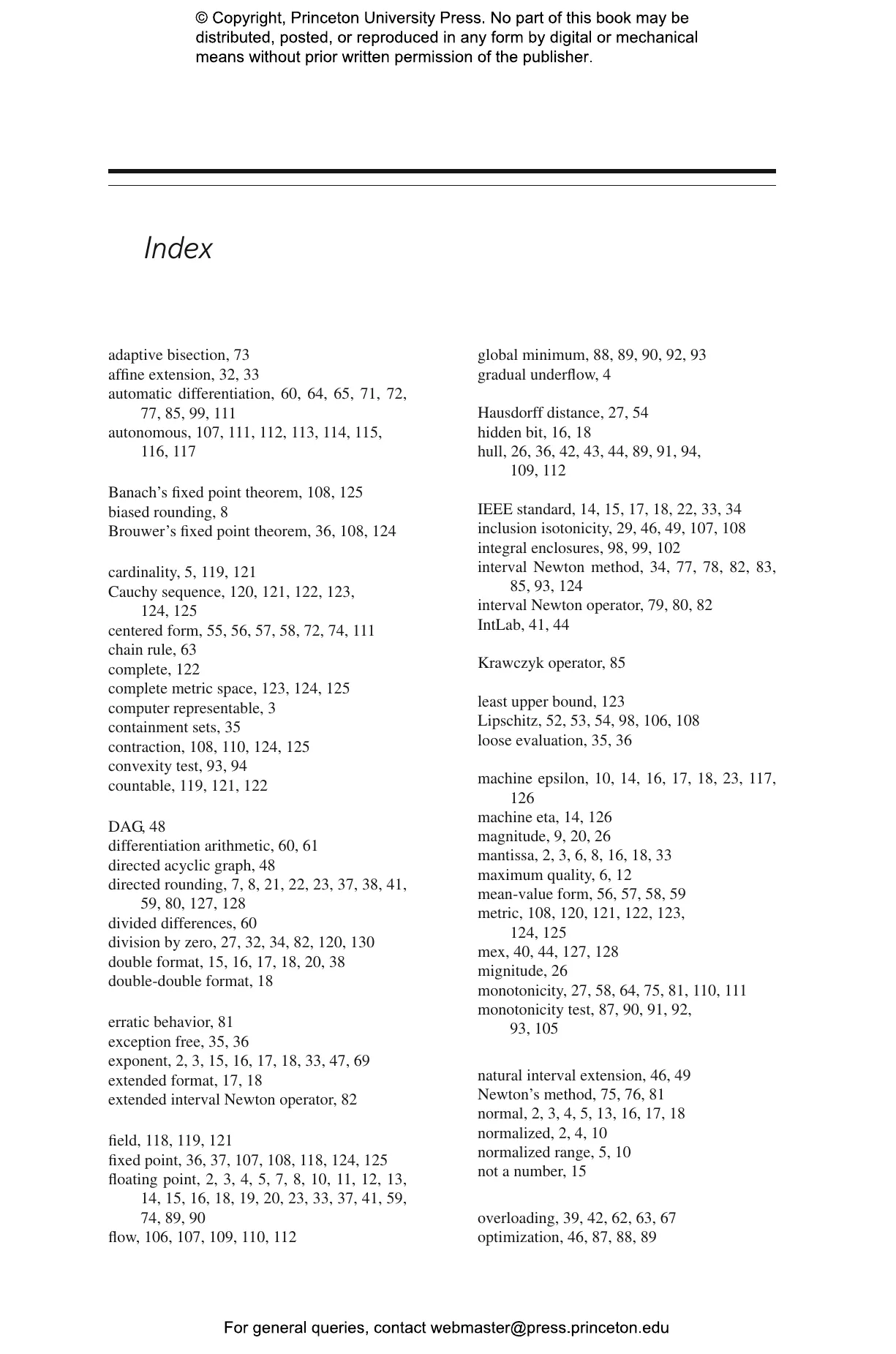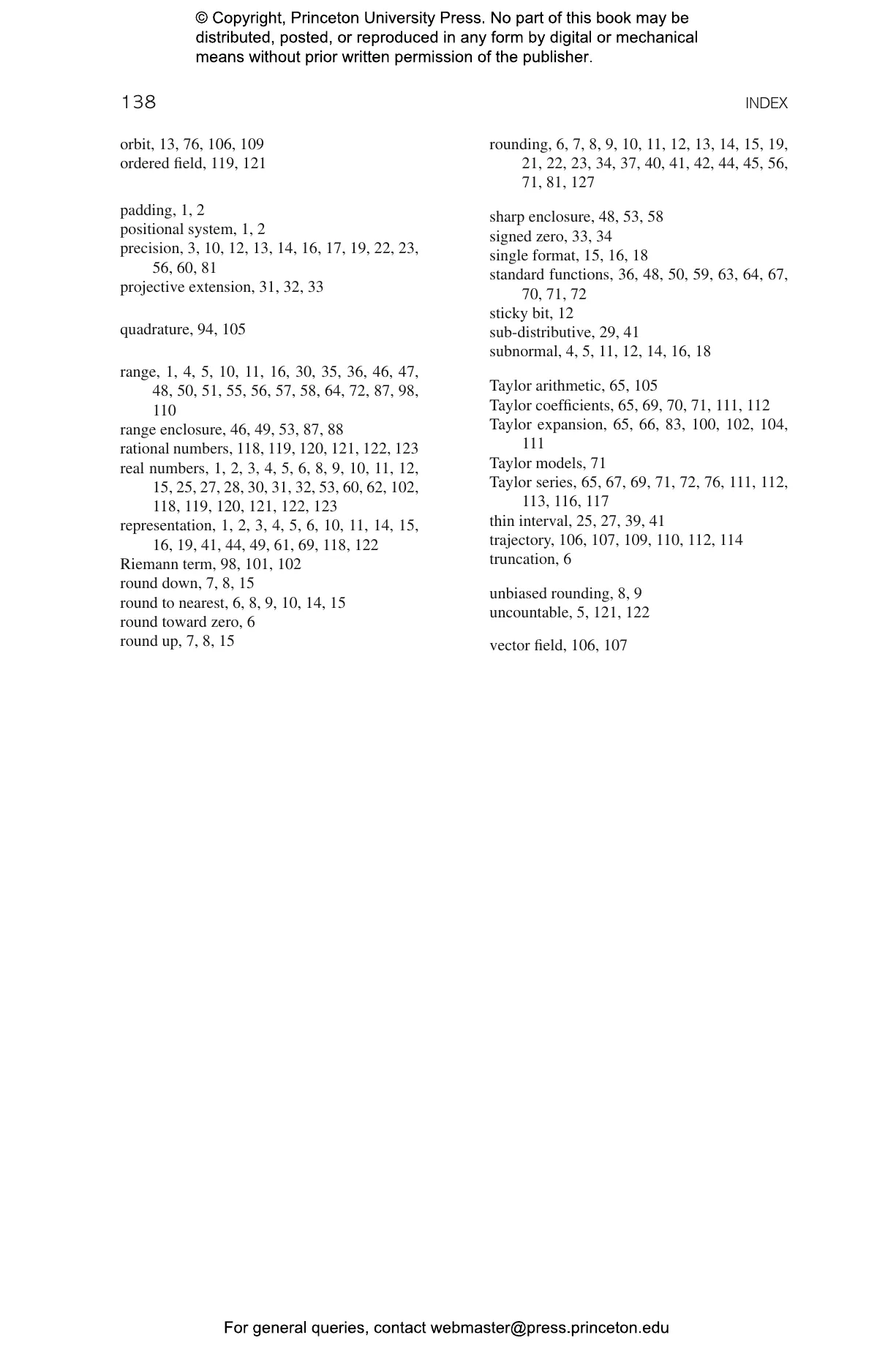Warwick Tucker

# Validated Numerics: A Short Introduction to Rigorous Computations

A comprehensive, self-contained primer on validated numerics## Paperback

Price:
\$45.00/£38.00
ISBN:
Published:
Jun 6, 2023
2011
Pages:
152
Size:
6.13 x 9.25 in.
Illus:
41 b/w illus. 12 tables.

This textbook provides a comprehensive introduction to the theory and practice of validated numerics, an emerging new field that combines the strengths of scientific computing and pure mathematics. In numerous fields ranging from pharmaceutics and engineering to weather prediction and robotics, fast and precise computations are essential. Based on the theory of set-valued analysis, a new suite of numerical methods is developed, producing efficient and reliable solvers for numerous problems in nonlinear analysis. Validated numerics yields rigorous computations that can find all possible solutions to a problem while taking into account all possible sources of error—fast, and with guaranteed accuracy.

Validated Numerics offers a self-contained primer on the subject, guiding readers from the basics to more advanced concepts and techniques. This book is an essential resource for those entering this fast-developing field, and it is also the ideal textbook for graduate students and advanced undergraduates needing an accessible introduction to the subject. Validated Numerics features many examples, exercises, and computer labs using MATLAB/C++, as well as detailed appendixes and an extensive bibliography for further reading.

• Provides a comprehensive, self-contained introduction to validated numerics
• Requires no advanced mathematics or programming skills
• Features many examples, exercises, and computer labs
• Includes code snippets that illustrate implementation